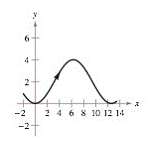Chapter 10.3, Problem 32E

Chapter
Section
Textbook Problem

Horizontal and Vertical Tangency In Exercises 31 and 32, find all points (if any) of horizontal and vertical tangency to the curve on the given interval. x = 2 θ y = 2 ( 1 − cos θ ) 0 ≤ θ ≤ 2 πTo determine

All the points of horizontal & vertical tangency to the curve on given interval.

Explanation

Given:

The provided figure is,

x=2θ&y=2(1cosθ) & 0θ2π

Calculation:

Differentiate both the functions with respect to θ,

dxdθ=2 & dydθ=2sinθ

For vertical tangency equate dxdθ=2

Still sussing out bartleby?

Check out a sample textbook solution.

See a sample solution

The Solution to Your Study Problems

Bartleby provides explanations to thousands of textbook problems written by our experts, many with advanced degrees!

Get Started